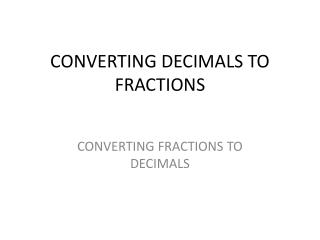DownloadDownload PresentationCONVERTING DECIMALS TO FRACTIONS

# CONVERTING DECIMALS TO FRACTIONS

Télécharger la présentation## CONVERTING DECIMALS TO FRACTIONS

- - - - - - - - - - - - - - - - - - - - - - - - - - - E N D - - - - - - - - - - - - - - - - - - - - - - - - - - -
##### Presentation Transcript

1. CONVERTING DECIMALS TO FRACTIONS CONVERTING FRACTIONS TO DECIMALS

2. Now students, This is an easy process. You may remember it from last year. Let’s review and practice converting decimals to fractions and fractions to decimals today.

3. Let’s start with changing a decimal to a fraction. • What is a decimal? • It is a number less than 1. • It is divided into units by tens. • What is .25? • Yes, twenty-five hundredths. • Can you write that as a fraction? • Twenty five hundredths • 25 over 100 – see how easy – now simplify • 25/100 = 1/4

4. Let’s practice some more.What strategy should you use?Place the decimal number as your numerator.The denominator starts with 1 and has as many zeroes as there are decimal places. .28 28 becomes the numerator the denominator begins with 1 And has as many zeroes as there are decimal places. 28 / 100

5. Your turn now. • .29 • .005 • .500 • 1.75 • 2.8 • .85

6. You are going to work with you’re the person next to you Seat 1 and 2 3 and 4 and so on. One of you will be the scribe and one will be the sage (Pinky and the Brain) I will give each team a deck of playing cards - One of the sage will deal two cards. These first two cards will make the decimal. The sage will tell the scribe to write the decimal number down. The sage will then convert the decimal to a fraction and tell the scribe what that fraction is. The scribe will write the fraction. You will now change roles and go again. We will do this until the timer goes off.

7. Now we are going to convert fractions to decimals. This is easy. Take the numerator and divide it by the denominator. 2/3 2 ÷3 3| 2 .66 2/5 2÷5 .4 Your turn 1/4 3/5 1/8 5/8 3/4 1/2 1-1/2 2-1/4

8. Now let’s return to Sage and Scribe practice. The sage will deal two more cards . The first card is the numerator. The second card is the denominator. The Sage will now convert the fraction to a decimal and tell the scribe what to write. Remember the scribe should be checking the work to make sure the Sage is doing it correctly. We will practice until the timer goes off.

9. Homework Page 414 3- 18 all Write fraction as a decimal. #3 1/2 1÷2 = .5 #4 3/4 #5 4/5 #6 7/10 Write the decimal as a fraction. #7 0.6 6/10 ÷2 = 3/5 #8 0.1 #9 0.95 #10 0.45

10. Find the product or quotient. #11. 82 x 0.64 #12 0.78 x 105 #13 15 ÷ 0.12 #14 58 ÷ 0.29 Solve the proportions. #15 1133 #16 a6 4 n 28 7 #17 25b #18 35 5 6 x 45